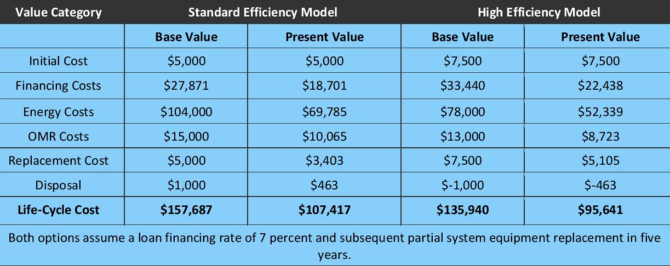## The Lifetime Value of Energy Efficiency

Convincing financial decision makers to invest in energy efficiency can be difficult because of the substantial upfront costs involved. Simple payback analysis often doesn’t help, since it ignores the benefits after the payback period — typically less than half the life of the project. It’s important to look beyond first cost and evaluate the costs and benefits of a project over its entire useful life. Lifecycle cost analysis (LCCA) does just that, estimating the lifetime financial impact of each project.

### The total cost of ownership

Lifecycle cost is the total cost of owning, operating, maintaining and disposing of equipment or building systems over the proposed lifetime of the project. If a new boiler has a projected lifetime of 20 years, that would be the analysis period.

Value categories incorporated in an LCCA include:

• Initial equipment purchase and installation
• Financing — loan payments and other financing charges
• Energy costs
• Non-fuel operating, maintenance and repair costs
• Disposal cost or residual value
• Equipment replacement costs

While the initial purchase price and financing costs are fixed, other costs can be more difficult to estimate. Calculate annual energy costs by multiplying the equipment nameplate (kW or Btuh) power rating, efficiency rating, operating hours and the average electric or gas rate. Estimating maintenance and repair costs over the life of equipment can be challenging. It’s also difficult to project how much it will cost to replace equipment in the future — use current costs as a starting point.

### The changing value of money

Money changes value over time. A dollar you’ll get 10 years from now is not worth as much today. An LCCA analysis converts future cash flows to their present value by discounting them with a discount rate. An appropriate discount rate is typically the weighted average cost of capital (WACC), which is the average return required by financing providers.

The WACC reflects both the current business risk of a company and the company’s financial risk (how it’s currently financed). An alternative for discount rate would be the marginal cost of capital, which is the cost of the last dollar of capital raised for the company. If financing is part of the project, use the loan interest rate as the discount factor.

### Lifecycle cost calculation

After identifying all costs by year and discounting them to their present day value, add them to determine total lifecycle costs:

LCC = I + F + E + OMR + D + R

Where:

• LCC = Lifecycle costs
• I = Initial costs
• F = Financing costs
• E = Energy costs
• OMR = Operating, maintenance and repair costs
• D = Disposal cost or value
• R = Replacement costs

The following table summarizes LCCA for two replacement options. The high efficiency option has higher initial and financing costs than the standard efficiency model. The initial costs are given in base value (the time of the initial investment), while future costs are discounted to present value using a discount rate of 7%.Since the total present value lifecycle cost of the high efficiency model (\$95,641) is less than that of the standard efficiency model (\$107,417), the high efficiency option is the more economical choice.

The economics of energy efficiency projects typically involve a great deal of uncertainty. An LCCA provides evidence that investing in energy efficiency saves more money in the long run. For more information on LCCA, see the Whole Building Design Guide.